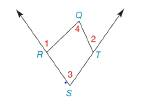Chapter 2.5, Problem 27E### Elementary Geometry for College St...

6th Edition
Daniel C. Alexander + 1 other
ISBN: 9781285195698

#### Solutions

Chapter
Section### Elementary Geometry for College St...

6th Edition
Daniel C. Alexander + 1 other
ISBN: 9781285195698
Textbook Problem
295 views

# Given: Quadrilateral RSTQ with exterior ∠ s at R and T. Prove: m ∠ 1 + m ∠ 2 = m ∠ 3 + m ∠ 4To determine

To prove:

m1+m2=m3+m4

Explanation

Given:

The following figure shows the given diagram.

Figure (1)

QRS is supplementary to 1 as shown in given figure.

QTS is supplementary to 2 as shown in given figure.

Approach:

The completed proof is shown in the following table.

 Proof Statements Reasons 1. The ∠QRS is supplementary to ∠1.The ∠QTS is supplementary to ∠2. 1. Given 2.m∠1+m∠QRS=180°m∠2+m∠QTS=180° 2. The sum of measures of two supplementary angles is 180° 3.m∠3+m∠4+m∠QRS+m∠QTS=360° 3. The sum of measures of four angles in a quadrilateral is,180×(4−2)=360° 4.m∠1+m∠2=m∠3+m∠4 4

### Still sussing out bartleby?

Check out a sample textbook solution.

See a sample solution

#### The Solution to Your Study Problems

Bartleby provides explanations to thousands of textbook problems written by our experts, many with advanced degrees!

Get Started

#### Find more solutions based on key concepts